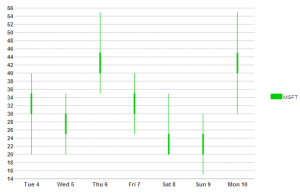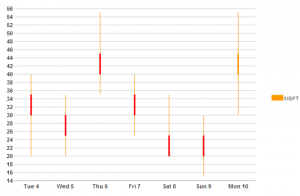﻿ Getting Started with Candlestick Chart For ASP.NET Wijmo# Getting Started with Candlestick Chart For ASP.NET Wijmo

In the 2014 v1 release we introduced Candlestick Chart.This chart can be used for data visualization in various financial applications, a common case for a candlestick chart is to show price movements of a stock. Let's see how we can use the new candlestick chart to show stock prices. We will start by creating a new project inside Visual Studio. Add a new class named "Stock", this is a simple class with properties for a stock.

``````public class Stock
{
public DateTime Date { get; set; }
public double Open { get; set; }
public double High { get; set; }
public double Low { get; set; }
public double Close { get; set; }
public int Volume { get; set; }
}
``````

We will need a collection of stock to add data to, we will name that "StockData"

``````class StockData:List<Stock>
{
public StockData()
{
this.Add(new Stock { Date = new DateTime(2014, 3, 4), Open = 35, High = 40, Low = 20, Close = 30, Volume = 2003343 });
this.Add(new Stock { Date = new DateTime(2014, 3, 5), Open = 30, High = 35, Low = 20, Close = 25, Volume = 2003343 });
this.Add(new Stock { Date = new DateTime(2014, 3, 6), Open = 45, High = 55, Low = 35, Close = 40, Volume = 2003343 });
this.Add(new Stock { Date = new DateTime(2014, 3, 7), Open = 35, High = 40, Low = 25, Close = 30, Volume = 2003343 });
this.Add(new Stock { Date = new DateTime(2014, 3, 8), Open = 25, High = 35, Low = 20, Close =20, Volume = 2003343 });
this.Add(new Stock { Date = new DateTime(2014, 3, 9), Open = 25, High = 30, Low = 15, Close = 20, Volume = 2003343 });
this.Add(new Stock { Date = new DateTime(2014, 3, 10), Open = 40, High = 55, Low = 30, Close = 45, Volume = 2003343 });

}
}
``````

Now place a C1Candlestick chart on a page. We can bind and style the chart in markup but lets do that using some C# code. We will create C1CandlestickChartBinding object where we will specify "Open", "High", "Low", "Close", also we can choose which type of chart we want to show, the options are "CandleStick", "OHLC", "HI".

`````` C1.Web.Wijmo.Controls.C1Chart.C1CandlestickChartBinding chartbinding=new C1.Web.Wijmo.Controls.C1Chart.C1CandlestickChartBinding();
chartbinding.HighField="High";
chartbinding.LowField="Low";
chartbinding.OpenField="Open";
chartbinding.CloseField="Close";
chartbinding.XField = "Date";
this.stockchart.Type = CandlestickChartType.Candlestick;
``````

Now lets bind the chart

`````` StockData data = new StockData();
this.stockchart.DataSource = data;
this.stockchart.DataBind();
``````

You can now run and view the chart.Lets add some style of our own to the series

``````            CandlestickChartStyle chartstyle = new CandlestickChartStyle();
chartstyle.HighLow.Fill.Color = Color.FromName("#ff9900");
chartstyle.FallingClose.Fill.Color = Color.FromName("#ff0000");
chartstyle.RisingClose.Fill.Color = Color.FromName("#ff9900");
That's it! Now run the project and view our changes, you should see the new styles applied.As we can see its simple to configure and get started with the new C1Candlestick chart. Download your copy of ASP.NET Wijmo.